• FIND_NDIM 沿维度查找第一个/最后一个非零元素索引。 I = FIND_NDIM(BW,DIM) 返回第一个非零的下标BW 沿维度 DIM 的元素。 输出 I 将包含零找不到非零元素元素位置 I = FIND_NDIM(BW,DIM,'first) 与 I = FIND_...
• a(x:end)表示取出a矩阵中第x个至最后一个元素 例如 a=[1:10] b=a(5:end) 执行结果 b=[5 6 7 8 9 10] 作为特例，取出a的最后一个元素可以使用 b=a(end) 执行结果 b=10 任意取出Array的倒数m个元素：Array(end-...
a(x:end)表示取出a矩阵中第x个至最后一个元素 例如 a=[1:10] b=a(5:end) 执行结果 b=[5 6 7 8 9 10] 作为特例，取出a的最后一个元素可以使用 b=a(end) 执行结果 b=10

任意取出Array的倒数m个元素：Array(end-(m-1):end) 例如： a=1:10; %提取最后5个元素，输出给b b=a(end-4:end) 输出为 b = 6 7 8 9 10
展开全文• 假如有一个向量 m = [ 1 2 0 3 4 0 5 0 0 0 0 0 0 0 0]，它的最后一个非零元素是5，它的索引（位置）为7。 ind = find(m,1,'last') 返回结果ind即为最后一个非零元素的位置（本例中为7）
假如有一个向量 m = [ 1 2 0 3 4 0 5 0 0 0 0 0 0 0 0]，它的最后一个非零元素是5，它的索引（位置）为7。
ind = find(m,1,'last')
返回结果ind即为最后一个非零元素的位置（本例中为7）
展开全文• ## MATLAB教程（1） MATLAB 基础知识

万次阅读 多人点赞 2017-10-26 20:57:32
初学。去年看过点点MATLAB，很久不用...第部分：MATLAB显示桌面的基本布局https://cn.mathworks.com/help/matlab/learn_matlab/desktop.html1.启动MATLAB后，桌面会显示默认布局，主要有以下三部分： 当前文件夹

初学。去年看过一点点MATLAB，很久不用，遗忘惊人。为了加深自己的印象，扎实基础，现将官网上的基础教程做简单的翻译。

首先，以下从九个部分简单介绍基础入门知识。
第一部分：MATLAB显示桌面的基本布局
https://cn.mathworks.com/help/matlab/learn_matlab/desktop.html
1.启动MATLAB后，桌面会显示默认布局，主要有以下三个部分：
当前文件夹命令行窗口工作空间
2.在MATLAB中一般需要创建变量和调用函数。
直接在命令行中输入变量名以及其值即可（比如：a = 1）,多个变量的创建同理，不再赘述。
需要注意的是，如果没有定义输出变量，MATLAB会使用一个名为ans的变量来储存计算结果。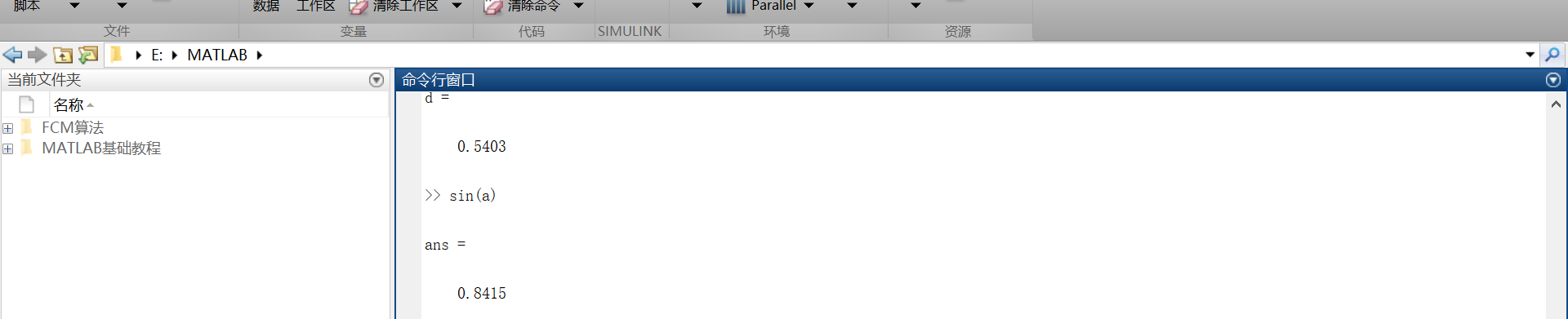3.如果语句以分号结束，表示MATLAB内部会执行这一运算，但是命令行不对计算结果进行输出。
第二部分：矩阵和数组
https://cn.mathworks.com/help/matlab/learn_matlab/matrices-and-arrays.html
大多数的编程语言一次性只能对一个数进行操作，而MATLAB主要被用来对整个矩阵或者数组进行操作。不管是什么类型的数据，所有的额MATLAB变量都是数组。矩阵是常用于线性代数的二维数组。
1.数组的创建
在一个单行中创建一个拥有四个元素的数组，其各个元素之间用逗号或者空格隔开。  例如：  a = [1 2 3 4]  这种数组叫做单行矩阵（行向量）。创建一个多行矩阵，其各个行之间用分号隔开。  例如：  b = [1,2;3,4;5,6]还可以用函数来创建矩阵。  例如：创建一个5行1列每一个元素都是0的列向量。  z = zero(5,1)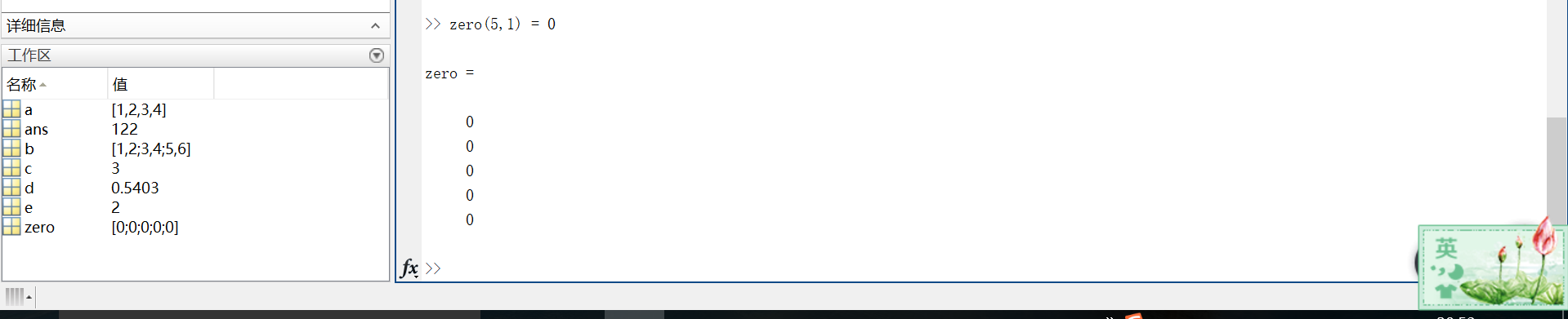2.矩阵和数组的操作
在MATLAB中，允许用一个单一的运算或者函数对矩阵中所有的数据进行处理（操作）。
例如：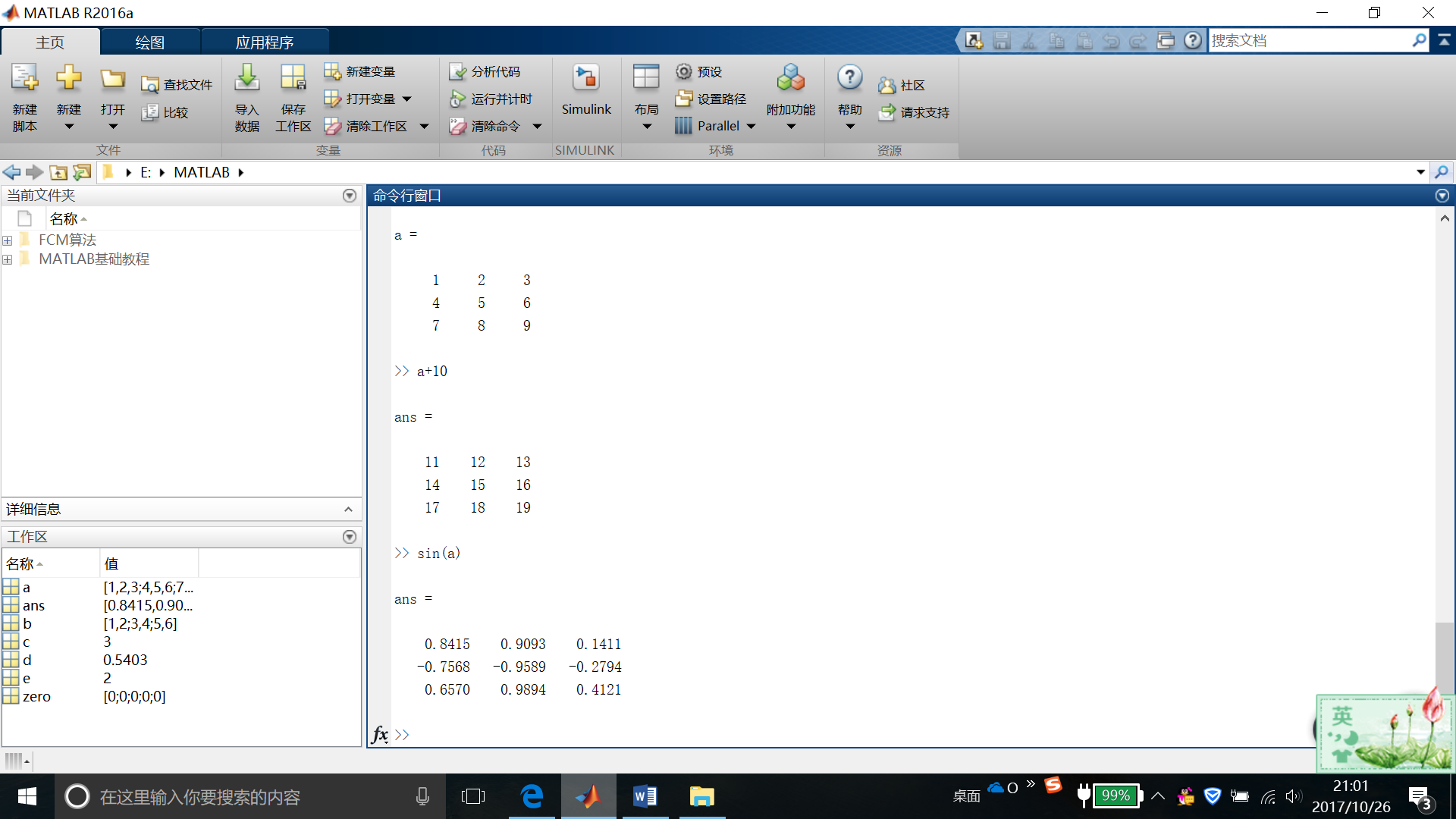如果要对矩阵进行转置，则使用单引号(‘)  例如：a’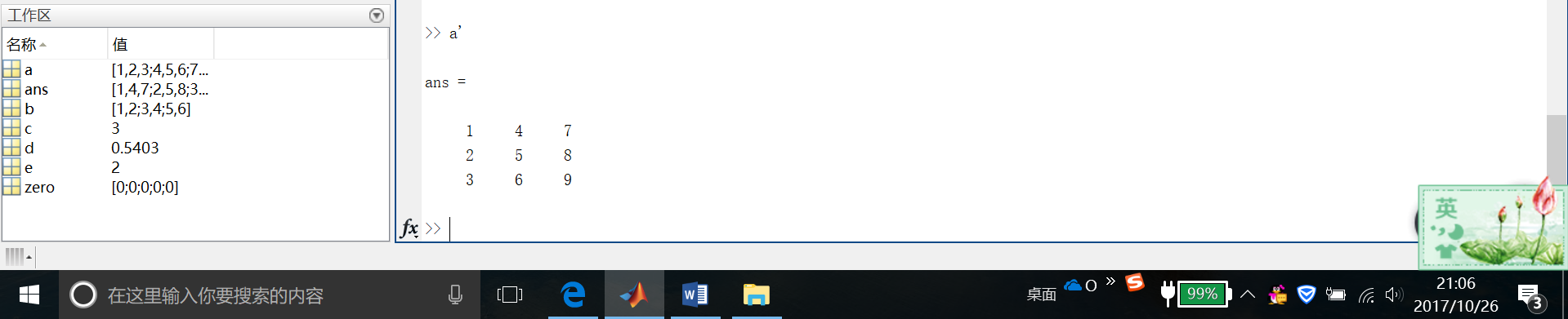可以通过执行标准矩阵乘法，通过*运算符来计算内积。
这是我自己的结果截图……很明显是不对滴~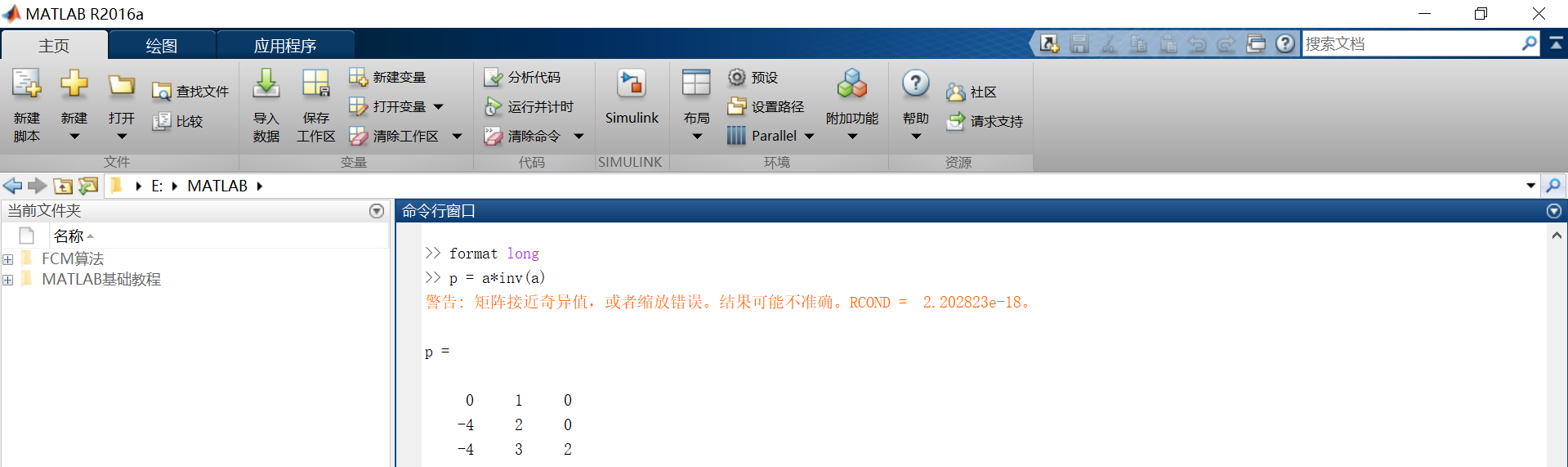然后下面是官网爸爸给的运行结果。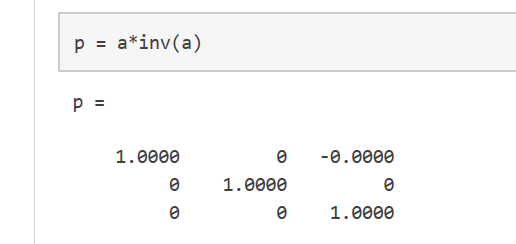我们都知道，一个矩阵与它的逆矩阵相乘的结果的确应该等于单位矩阵。至于这里为什么不对，还希望各位大佬们能不吝赐教，大恩不言谢~
这里要注意的是，p不是一个整数值矩阵，MATLAB存储数字是以浮点型存储的，真实值和它的浮点数在运算时有小小的不同，我们可以使用格式化命令，显示更多的小数位数。

比如：  format long  p = a*inv(a)

可以使用format short来重置到较短格式的使用。
x = a.*a用来表示对矩阵的每一个元素做平方操作。  若需要对每一个元素做立方运算，则用x = a.^3(如图)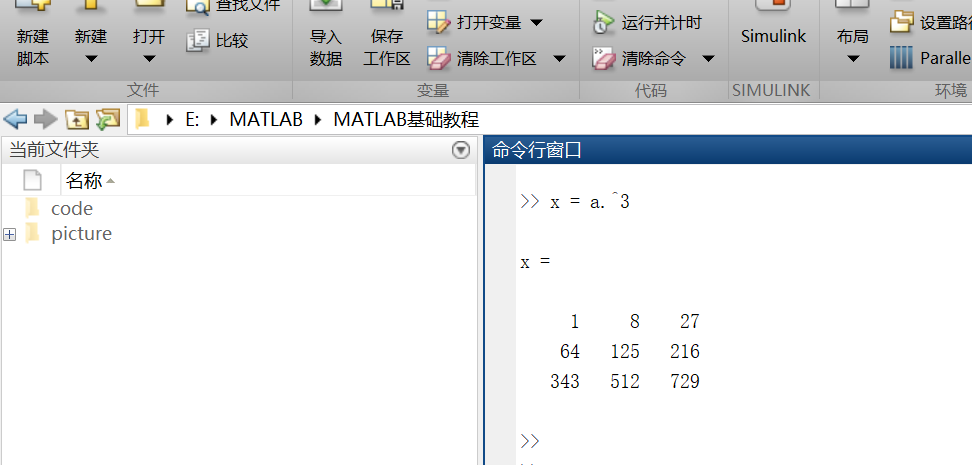矩阵的扩充  行方向上：A = [a,a]  列方向上：A = [a; a]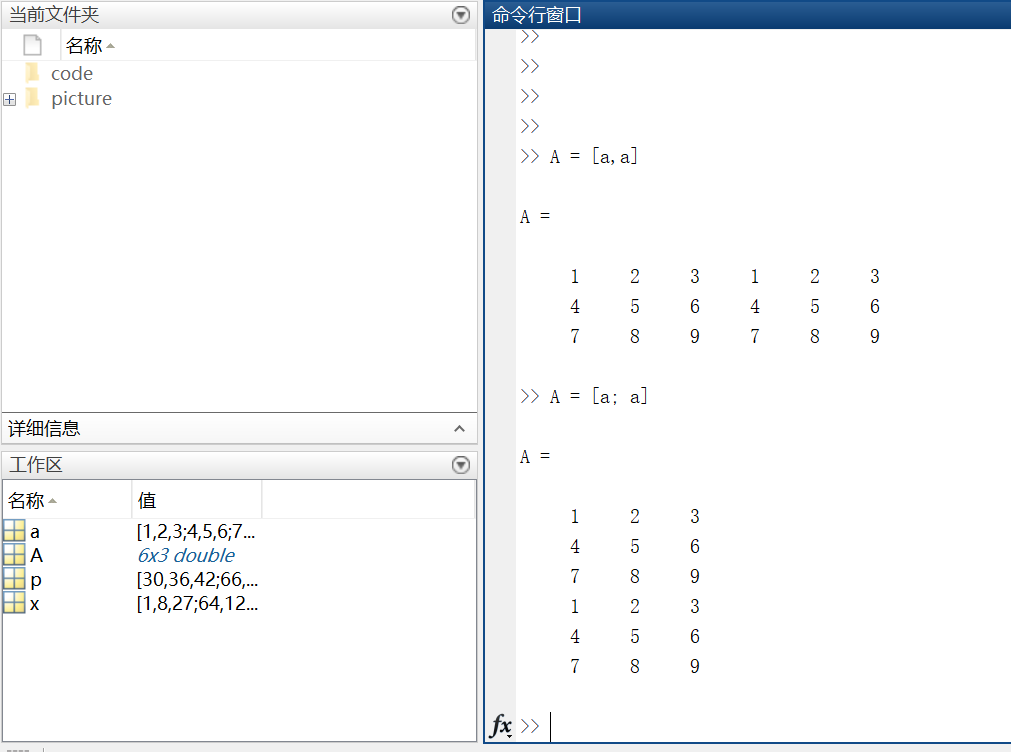复数  复数由实部和虚部组成，它的虚部是-1的平方根。  即sqrt(-1) = 0.0000 + 1.0000i  一般用i或者j来代表复数的虚部部分。
第三部分：数组的索引
https://cn.mathworks.com/help/matlab/learn_matlab/array-indexing.html
MATLAB中的每一个变量都是可以存储多个值的数组。如果想要使用数组中的某一个值，则需要用到索引。
1.单个元素的索引
从一个数组中索引某个元素一般有两种方法：  第一种比较常见：数组名加要索引的元素所在的行和列，例如A(2，4) = 8。  第二种不太常用：数组名加一个下标，该下标按列遍历。A(14) = 8。【这里不太好表达，上图吧~】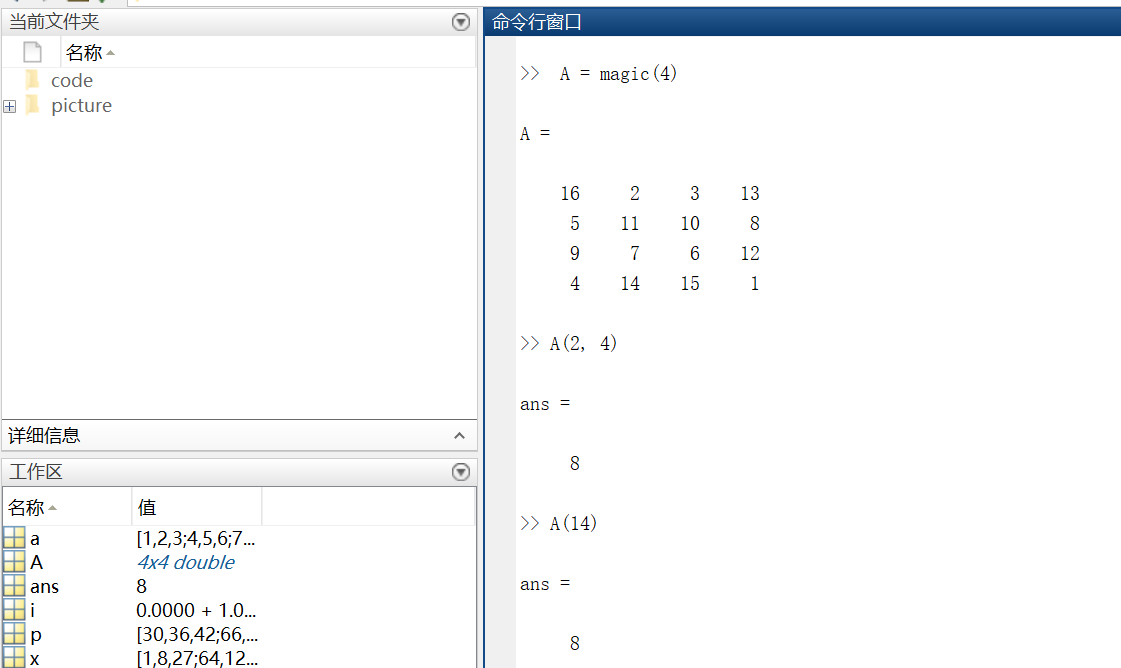再多说一句，其实A(14)中的14表示按列数第14个元素！  利用下标索引元素这种方式叫做线性索引。  注意：如果索引超出矩阵维度，MATLAB会报错。
2.多个元素的索引  引用数组的多个元素，使用冒号操作符m : n(m，n分别表示索引开始和结束的范围)
例1索引第二列第1个元素到第3个元素。  例2索引第三行全部元素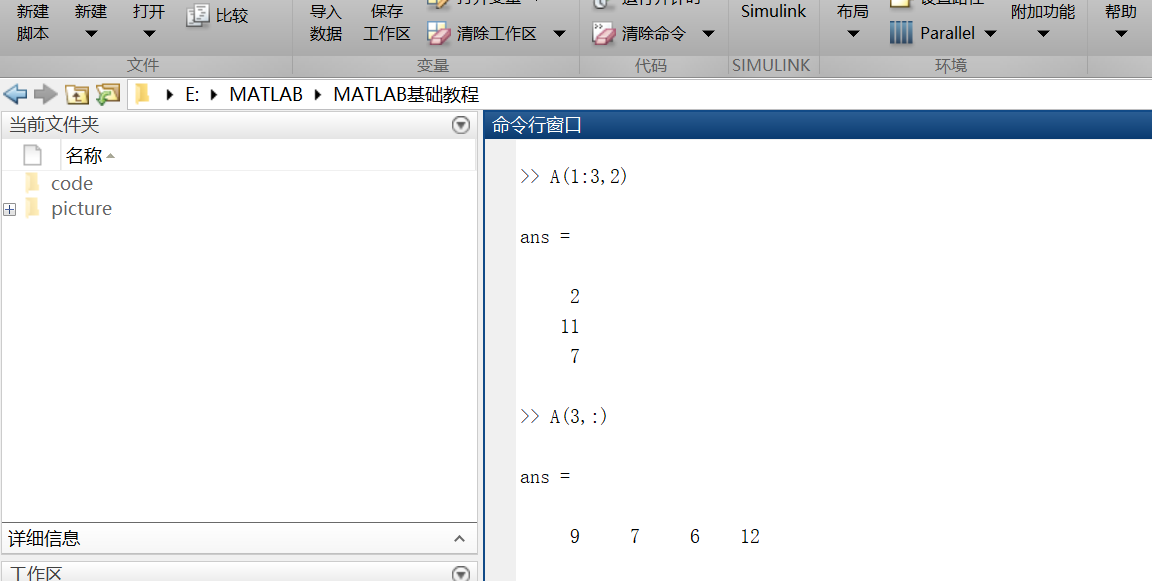不难发现，索引行则将行数放在逗号前；索引列要将列数放在逗号后。
另外，冒号运算符也可以帮助创建一组等距的矢量值，例如：  B = 0:10:100  —->B = 0 10 20 30 40 50 60 70 80 90 100  输出一个从0到100步长为10的数组。
第四部分：工作空间变量
https://cn.mathworks.com/help/matlab/learn_matlab/workspace.html
工作区包含你创建的变量或者从数据文件或其他程序导入到MATLAB中的变量。例如，我现在创建两个变量 ：  A = magic(4)  B = rand(3,5,2)
我们可以通过whos来看工作空间的内容。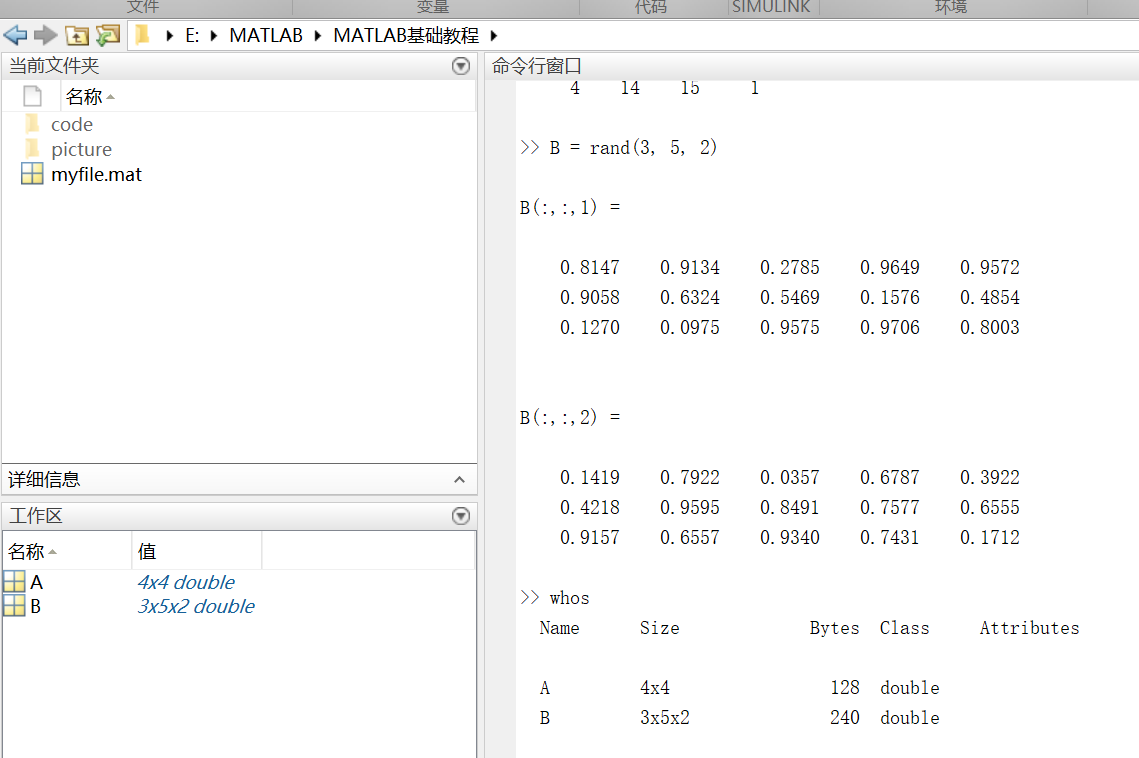第五部分：文本和字符
https://cn.mathworks.com/help/matlab/learn_matlab/character-strings.html
在处理文本时，将其中的字符序列用单引号括起来，可以将文本分配给变量。  例如：myText = ‘Hello, world’;如果文本本身包括一个单引号，则在定义时使用两个单引号。  例如：otherText = ‘You”re right’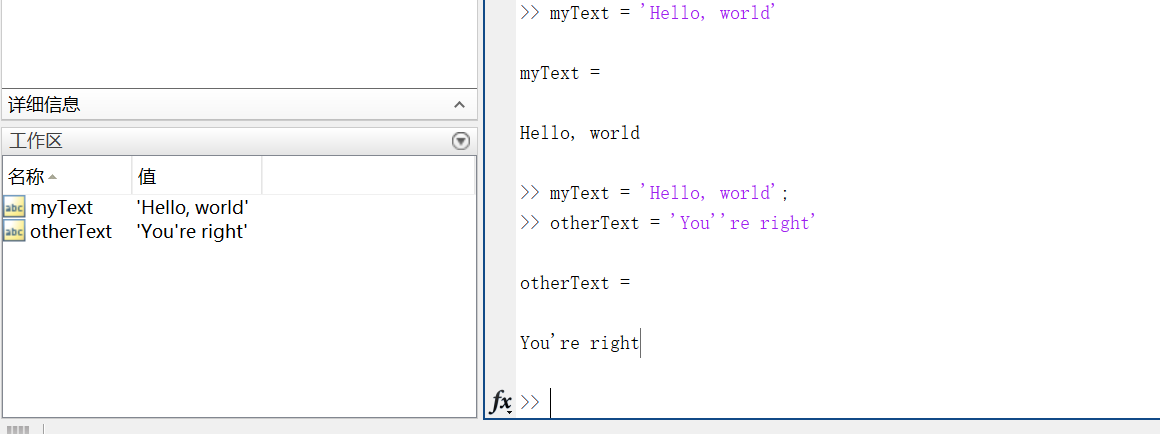同所有的MATLAB变量一样，上述创建的两个变量也都是数组，他们的数据类型是字符型。注意：字符数组也可以像数字数组那样用方括号括起来表示。
要将数字转换成字符，就要用到函数。例如num2str()或者int2str()。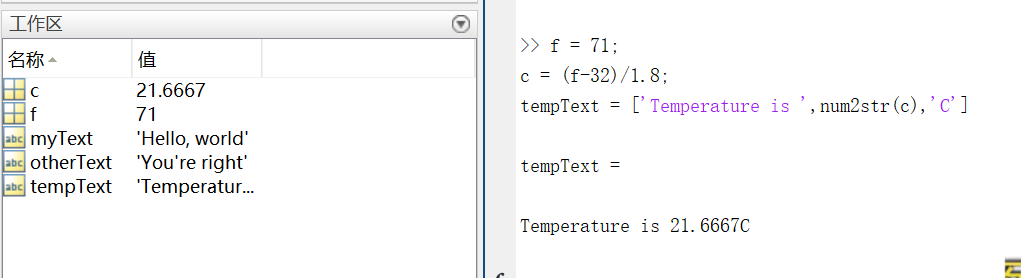第六部分：函数调用
https://cn.mathworks.com/help/matlab/learn_matlab/calling-functions.html
MATLAB提供了大量函数来执行计算任务，函数相当于其他编程语言中的子程序或方法。  例如求最大值的函数，max()。
将输入参数用括号括起来，如果有多个参数，则将参数用逗号隔开。  一般通过将函数值赋给某个变量这种方式来返回（输出）函数值，如果有多个输出参数，则需将它们用方括号括起来。  例如：  A = [1 3 5];  [maxA,location] = max(A)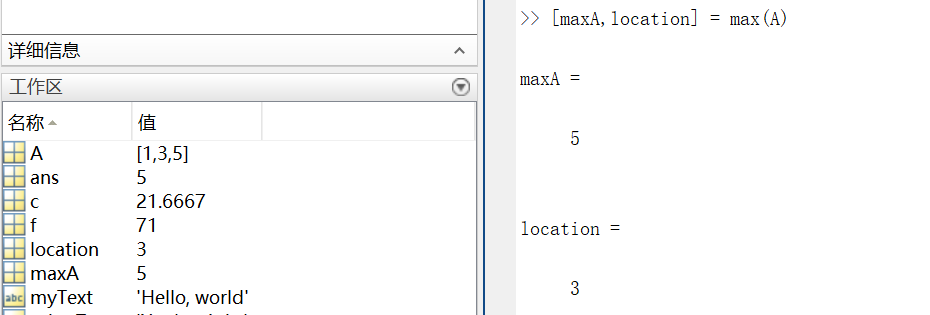可以用单引号括住任何字符进行输入。  例如：  disp(‘hello world’)  ===>输出 hello world

disp()用来显示变量的内容,括号里可以是字符,结构体,矩阵等等，类似于c语言的printf()。

clc函数被用来清空命令行。
第七部分：二、三维图
https://cn.mathworks.com/help/matlab/learn_matlab/plots.html
折线图 （1） 画图 x = 0:pi/1000:2*pi;  y = sin(x);  plot(x,y)
这里x就用到了前面说到的索引。x表示0到2*pi之间步长为pi/100的值。  二维图如下：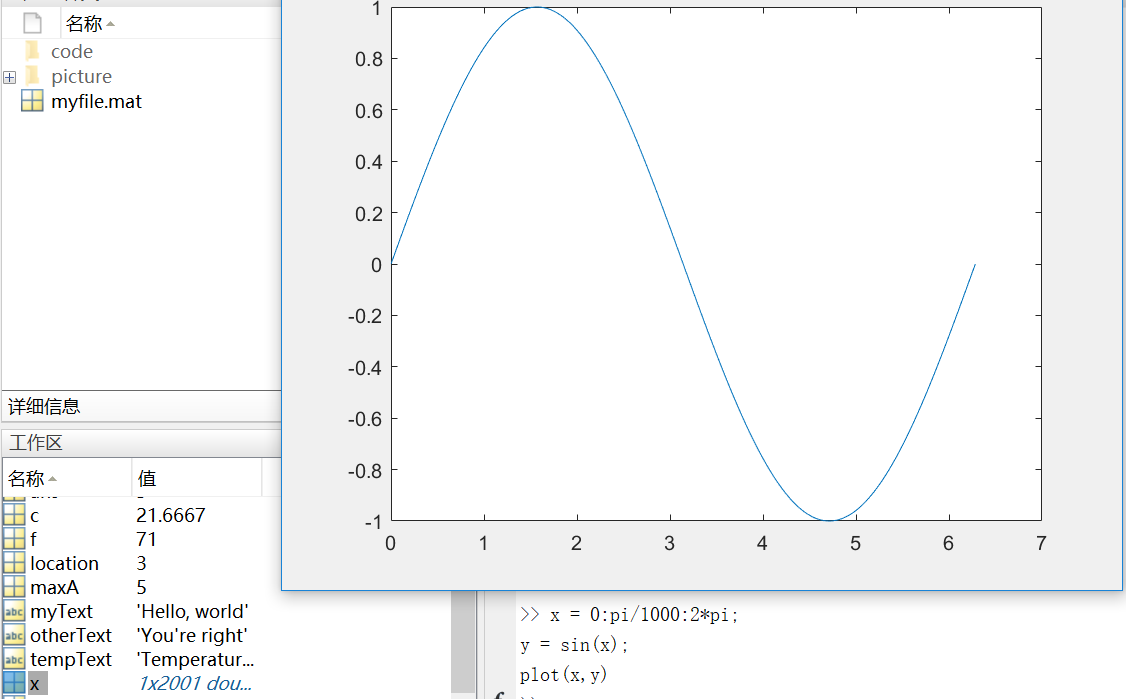这里多说一下步长，步长决定了横轴取值的密集程度。下图是将步长改为pi/10之后的图像。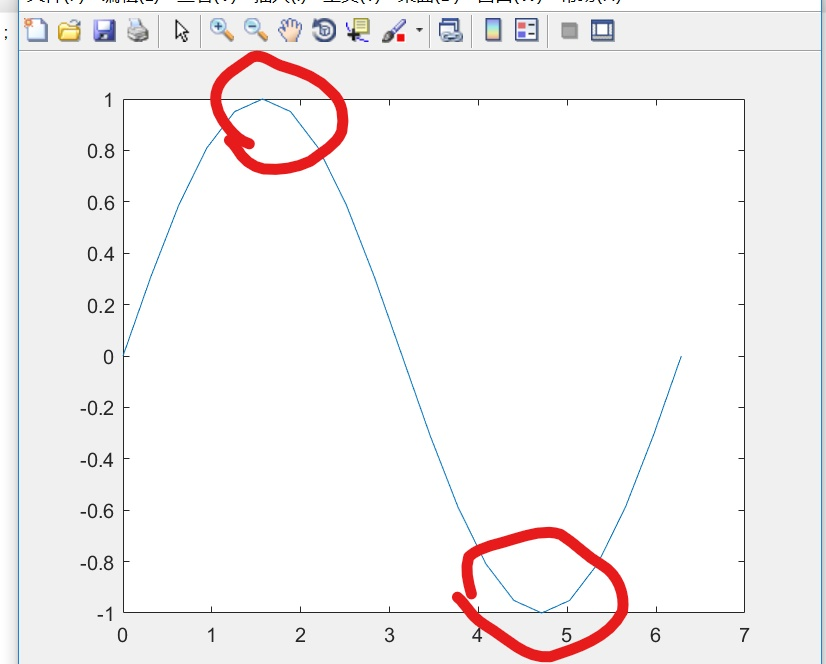对比图中标记处不难发现，步长增大，图像的精确度变低，所以这里尽量保证步长足够小，使得x可以取到足够多的值
（2） 坐标  我们可以对横轴和纵轴分别命名，并对上述正弦图像加上标题。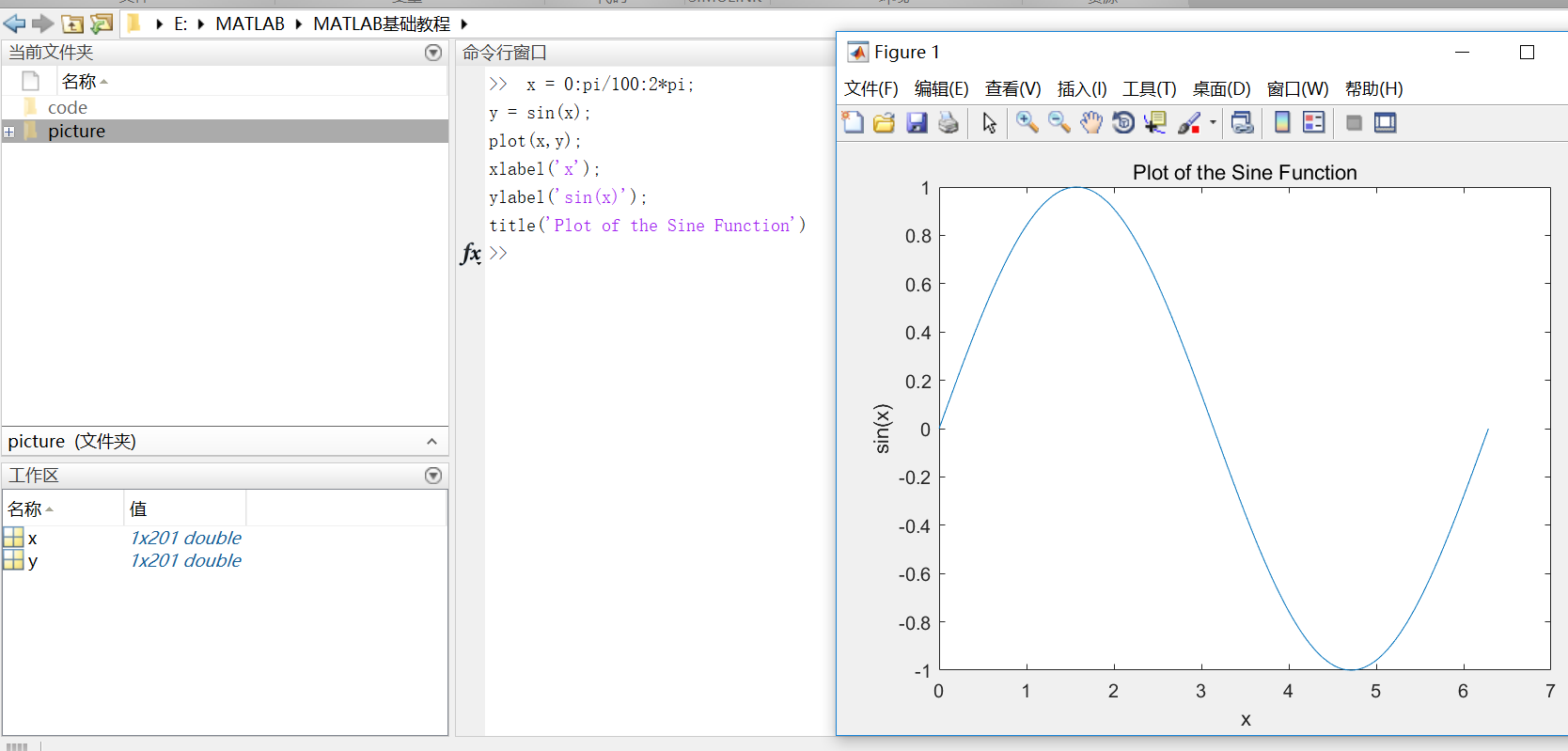（3）plot(x, y, ‘r–’)  通过在图像函数中添加第三个输入参数，可以用红色虚线绘制与原来相同的变量。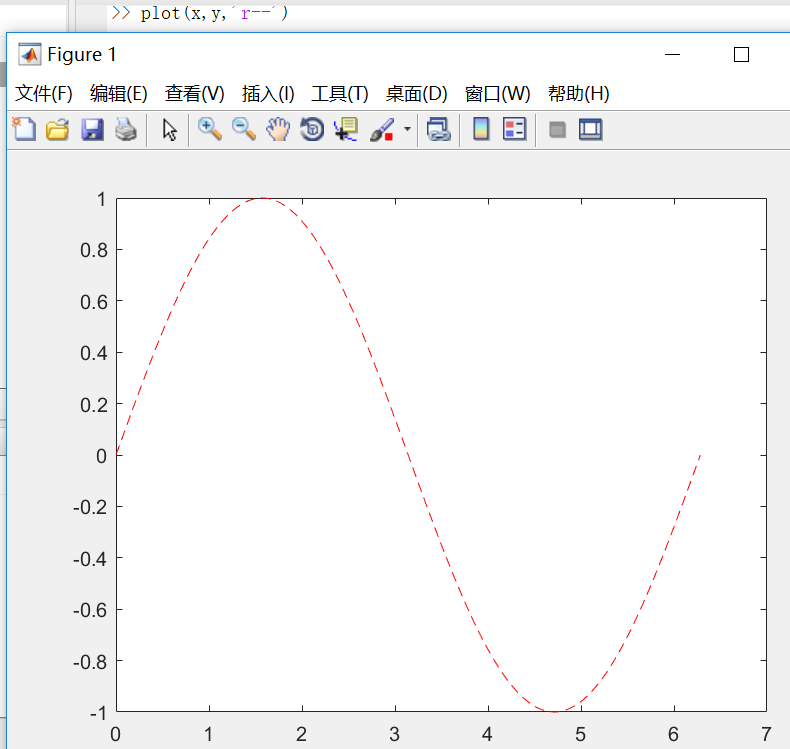其中第三个参数规定了线条的颜色、样式和标记的字符。（例如上例中的‘r–’表示红色虚线。）标记是指对每一个数据点用参数所给的标记形式进行表示。（例如’g:o’表示用‘o’连成一条绿色的线输出=.=我这个解释没谁了，讲不清楚还是上图吧…..）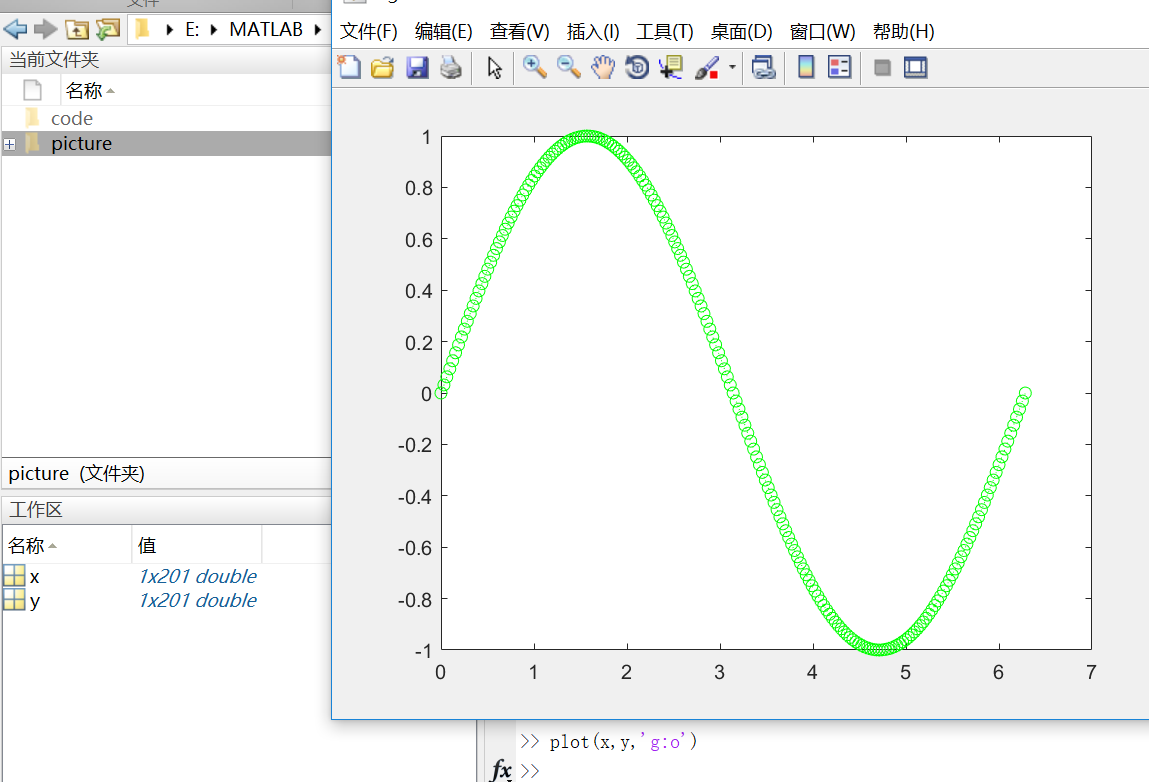这样的标记除了’o’，还有‘+’，‘*’等等。
这时候你会发现，我们开始为第一个图定义的标题和标签不再显示在当前图形窗口中了。这是因为在默认情况下，重新调用一个绘图函数，MATLAB就会清除数据，重置轴和其他元素来准备新的绘图。  若要将图添加到现有图形，使用hold命令即可。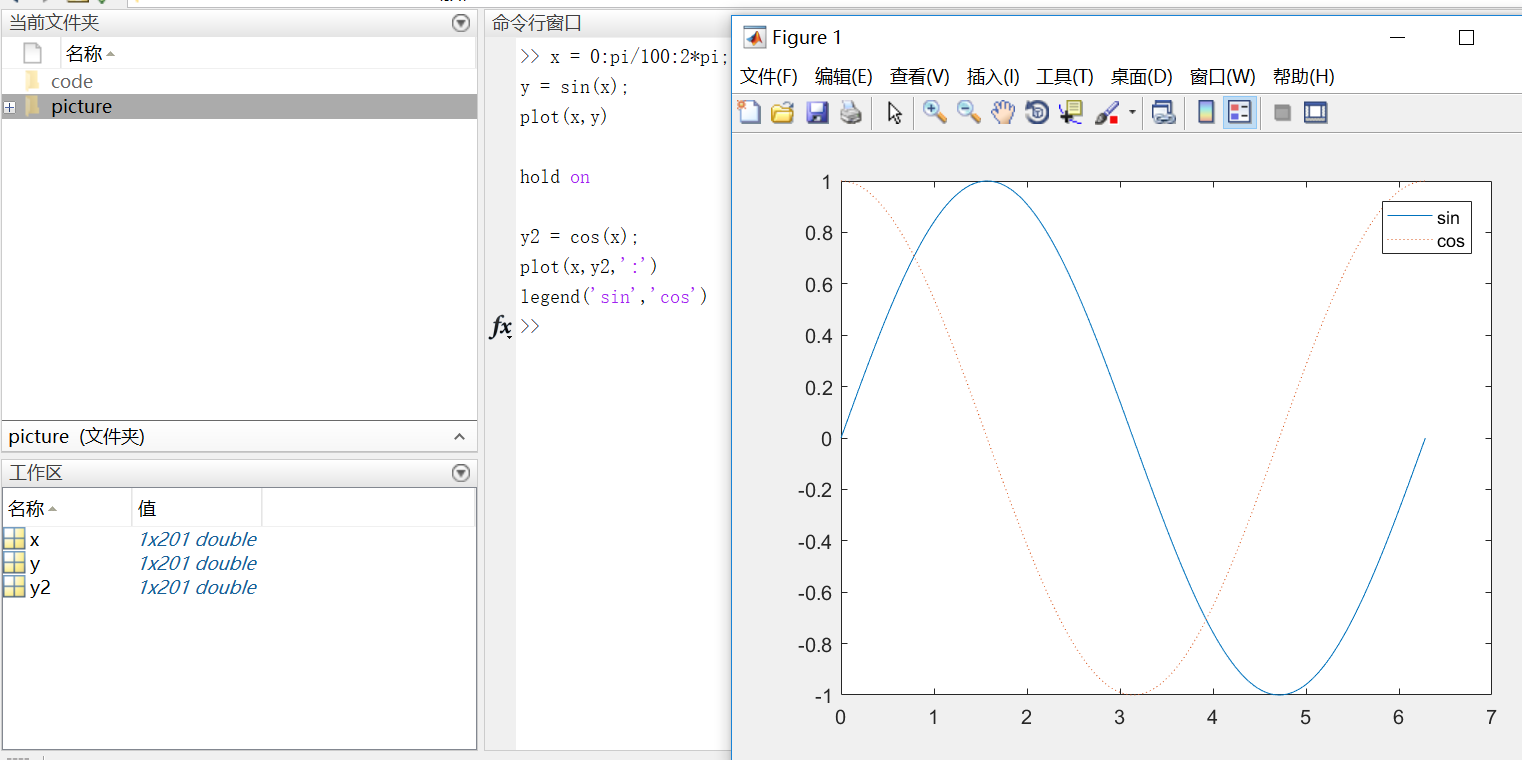在使用hold off或close the window命令之前，所有的图都会出现在当前的图形窗口中。
3D图像 3D图像通常用有两个参数的函数来定义一个曲面，z = f(x,y) 。  对于z，首先使用meshgrid命令在函数定义域内创建若干(x,y)。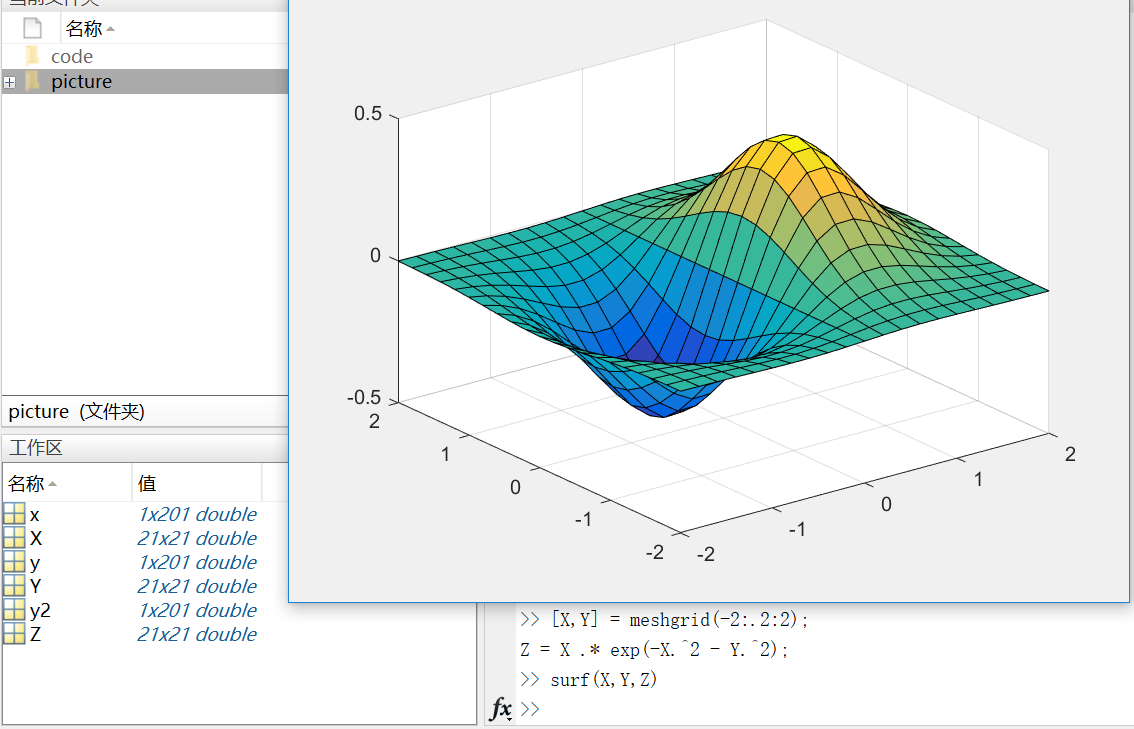这里需要注意的是，在matlab中，.2代表0.2  (-2:.2:2)表示在-2到2的区间上以0.2的长度为间隔的向量；
当变量x是由向量、数组或隔开的点组成（如x=0：0.1：1）时，则后面有x变量的方程要加点 (.)，例如：y=x.*exp(-x.*2)  但是如果x是连续的变量，如syms x，则方程写成y=x*exp(-x*2)。
Subplots  你可以用subplot函数在同一窗口的不同区域显示多个图像。  subplots函数的前两个输入参数显示当前窗口每行每列的图像数量，第三个输入参数指定活动区域。例如，在图形窗口创建四个区域来分别输出图像。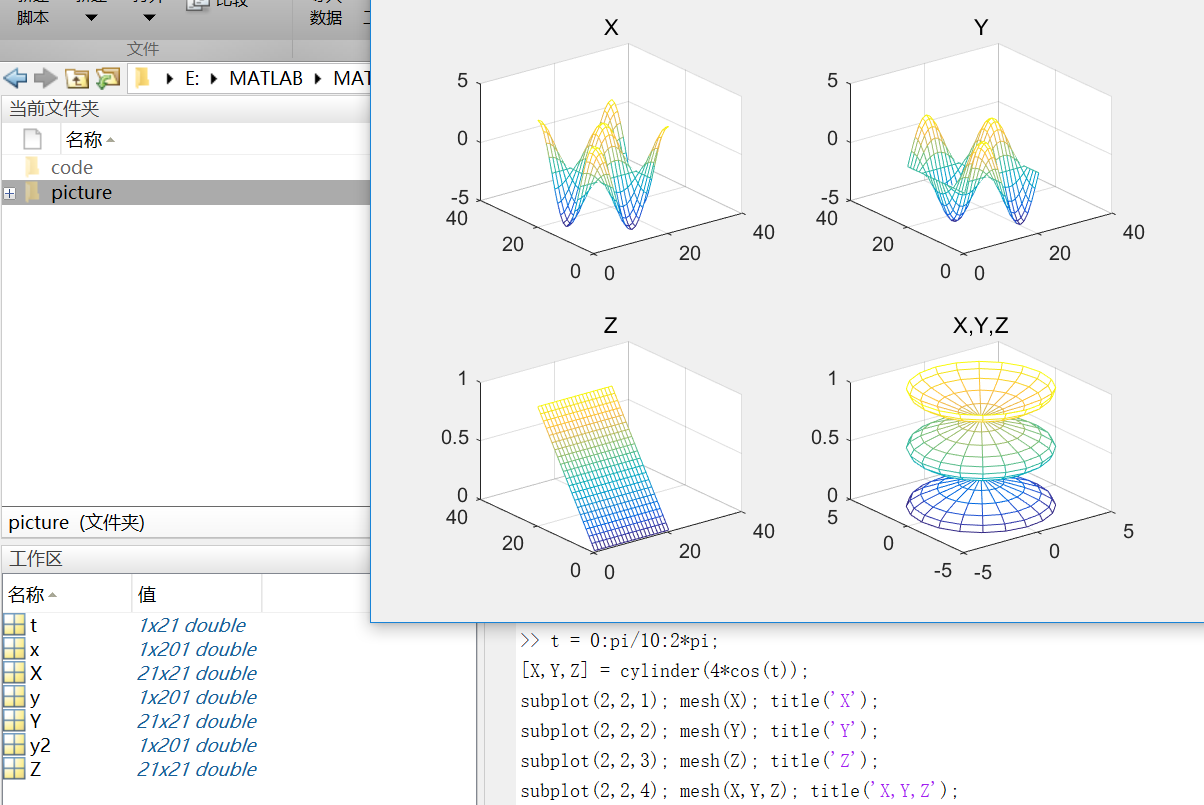mesh()生成由X，Y和Z指定的网线面，由C指定的颜色的三维网格图。
第八部分：程序和脚本
https://cn.mathworks.com/help/matlab/learn_matlab/scripts.html
示例脚本（从均匀分布中生成随机数据并计算平均值。绘制数据和平均值。）  用edit命令来创建脚本：  edit plotrand  这样就打开了一个叫plotrand.m的空文件，输入产生随机数向量的代码：
n = 50;
r = rand(n,1);
plot(r)

然后，添加代码在图中绘制水平线：
m = mean(r);
hold on
plot([0,n],[m,m])
hold off
title('Mean of Random Uniform Data')

在写代码过程中，可用（%）在代码之间添加注释。  最后保存脚本，在命令行窗口输入plotrand运行脚本。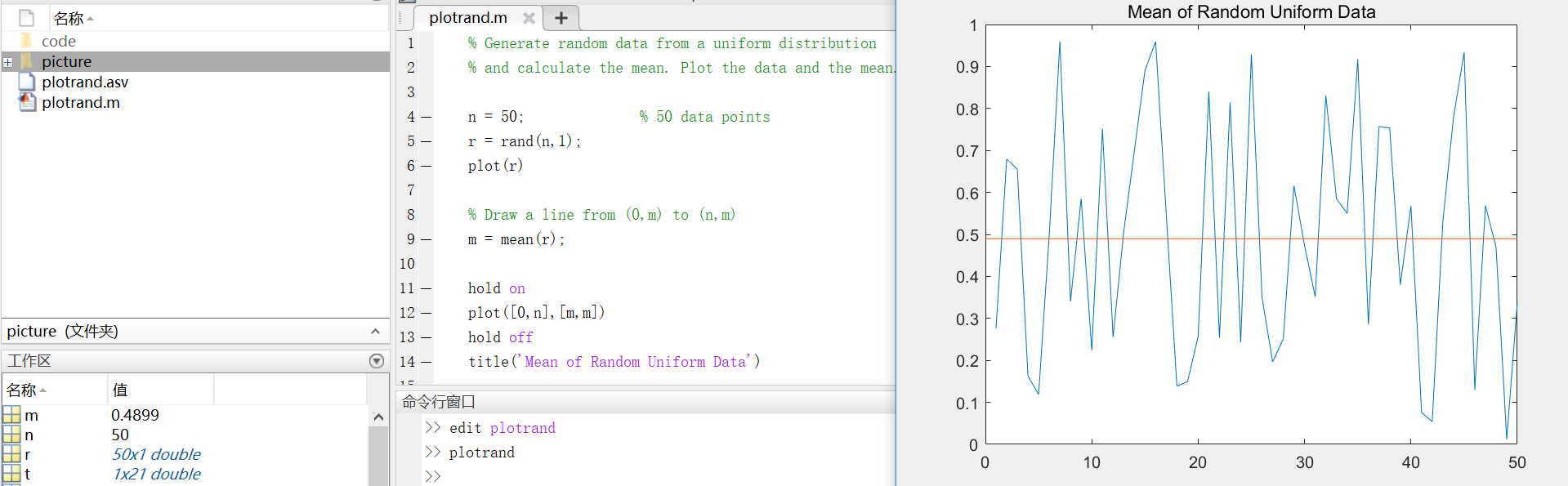循环和条件语句  在脚本中，可以循环使用部分代码，并且通过使用（for,while,if,switch等关键字）来执行这部分代码。  例如，创建一个叫calcmean.m的脚本，利用for循环计算五个随机样本的平均数和总平均数。
nsamples = 5;
npoints = 50;

for k = 1:nsamples
currentData = rand(npoints,1);
sampleMean(k) = mean(currentData);
end
overallMean = mean(sampleMean)
现在，修改for循环，以便每次迭代时都可以查看结果。在运行脚本时，会先显示中间结果，再计算总体均值。
for k = 1:nsamples
iterationString = ['Iteration #',int2str(k)];
disp(iterationString)
currentData = rand(npoints,1);
sampleMean(k) = mean(currentData)
end
overallMean = mean(sampleMean)

calcmean
在编辑器中，在calcmean.m的最后添加条件语句，使它根据不同的总体均值返回不同的信息。
if overallMean < .49
disp('Mean is less than expected')
elseif overallMean > .51
disp('Mean is greater than expected')
else
disp('Mean is within the expected range')
end
脚本位置  MATLAB中，要运行脚本，文件必须位于当前文件夹或搜索路径中的文件夹中。  默认情况下，MATLAB安装程序创建的MATLAB文件夹位于搜索路径上。如果要在另一个文件夹中存储和运行程序，需要将其添加到搜索路径中：在当前文件夹浏览器中选择文件夹，右键单击，然后选择“添加到路径”。
第九部分：帮助和文档
https://cn.mathworks.com/help/matlab/learn_matlab/help.html
使用doc命令在单独的窗口中打开函数文档。 doc mean键入左圆括号后暂停，MATLAB在命令窗口中就会显示函数提示（函数文档的语法部分）。 mean(使用帮助命令后，在命令窗口中查看函数的简单用法概括。 help mean
以上就是MATLAB的基础入门知识，欢迎指正呦~
展开全文• NANFILLTS(..., NOTRAIL) 为了避免推断 a 的最后一个值时间序列直到面板结束，设置NOTRAIL 为真 (1)。 默认情况下为假 (0)。 TSPANEL = NANFILLTS(...) 返回填充 NaN 的相同矩阵。 注意：领先的 NaN 保持不变。
• 会创建一个包含A中第 2 行上所有元素的行向量。 任务：创建一个名为density的变量，其中包含名为data的矩阵中第 2 列上的所有元素。 (2)冒号运算符可以引用某个值范围。以下语法会创建一个包含矩阵A的第 1 行、第 2...

(1)用作索引时，冒号运算符 (:) 可指代该维度中的所有元素。以下语法
x = A(2,:)

会创建一个包含 A 中第 2 行上所有元素的行向量。
任务：创建一个名为 density 的变量，其中包含名为 data 的矩阵中第 2 列上的所有元素。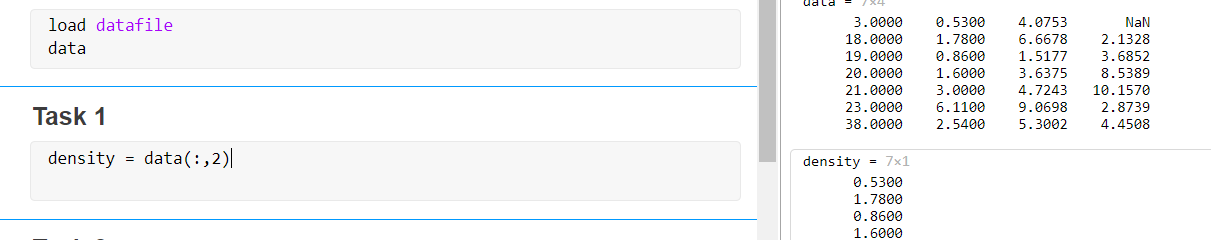(2)冒号运算符可以引用某个值范围。以下语法会创建一个包含矩阵 A 的第 1 行、第 2 行和第 3 行所有元素的矩阵。
x = A(1:3,:)
任务：创建一个名为 volumes 的变量，其中包含 data 的最后两列的所有元素。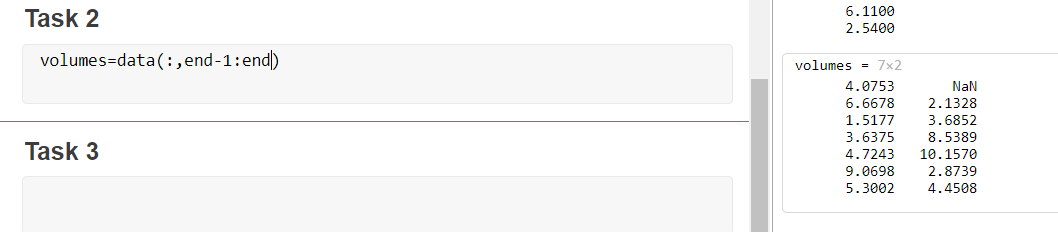(3)单个索引值可用于引用向量元素。例如
x = v(3)



展开全文机器学习 索引 数据结构 python
• 1.向量后面加元素 >> x=[1 2 3 4 5 ]; >> y=6; >> x=[x y] x= 1 2 3 4 5 62.构建矩阵
• G=[1,2,3;4,5,6;7,8,9];删除第三行G(3,:) = []; 删除第3列G(:,3) = [];
• 1 取出向量中下标对应的元素 格式：a(i) 2 取向量中最大的元素 格式：max(a) ...格式：a(i:j)，取第i到第j连续的元素 5 向量的长度 格式：length(a)或numel(a) 6 对向量中所有元素求和 eg：sum(a) ...矩阵
• 、变量 变量是保存数据信息的最基本的数据类型。变量的命名应遵循如下规则： 变量名必须以字母开头 变量名由字母、数字和下划线混合组成 变量名区分字母大小写 MATLAB有一些特定含义的默认变量，可以直接使用...数值计算 基础 语言 笔记
• 删除数组 arr 最后一个元素。不要直接修改数组 arr，结果返回新的数组 示例1 输入 [1, 2, 3, 4] 输出 [1, 2, 3] 1.利用数组的length function truncate(arr) { var newarr=arr.slice(0); newarr....
• 一个矩阵中所有元素的平均值   在学均值滤波的时候，需要用到求一个矩阵所有元素的平均值，学习C++的我居然写了个循环，先算总值，再除以个数.(现在想想当时自己好笨！)   先上代码吧(用...
• * 获取array中的最后一个元素 * Gets the last element of array * @param {Array} array The array to query * @returns {*} Returns the last element of array * @example * last([1, 2, 3]) * // =&...
• 如果要向已知的矩阵中添加列，如下 a=[] for i=[1:0.0001:10] a=[a,i]; end 向已知的矩阵中添加行，如下 a=[] for i=[1:0.0001:10] a=[a;i]; end
• [BP]=numunique(A) 对于数组 A 返回与 A... 请注意，P 的每个单元格都列出了 A 的所有索引，而不仅仅是第一个最后一次出现，具有重复值。 这与 Mathwork 的不同功能唯一。 有时我们需要知道 A 具有相同值的所有索引。
• 其中表达式是用运算符将有关运算量连接起来的式子，其结果是一个矩阵 例如计算表达式的值，并显示计算结果，在MATLAB命令窗口输入指令： 其中pi和i都是MATLAB预先定义的变量分别代表圆周率π和虚数单位。 2....
• ## matlab人脸识别论文

万次阅读 多人点赞 2019-10-11 17:41:51
本文设计了种基于BP神经网络的人脸识别系统,并对其进行了性能分析。该系统首先利用离散小波变换获取包含人脸图像大部分原始信息的低频分量,对图像数据进行降维;再由PCA算法对人脸图像进行主成分特征提取,进--步...
• >> mn{1}=[1 2] mn = [1x2 double] >> mn{2}=[1 2 3] mn = [1x2 double] [1x3 double] >> mn{3}=[1 2 3 4] ... [1x2 double] [1x3 double] [1x4 double]>... [1x3 double] [1x4 doubl...
• 之前一直在想取最后一个不就是获取数组的长度-1，从而获取最后一个元素的键名吗？ 但是现在想想 如果键名是字符怎么办？ 这样就取不到了。 PHP中就有一个函数可以解决这个问题end($array) 当然用array_pop($...
• 由于Matlab是按照列优先存储数据，...基本思想：每列5个元素对应的列select_minQ-1乘以5，计算每列最后一个元素的坐标位置； #由于matlab没有index=0； select_minQ-1表示最后一个元素位置，加上[1;2;3;4;5]，表示...离散数据
• ## 详解遗传算法（含MATLAB代码）

万次阅读 多人点赞 2019-05-29 11:30:47
目录 、遗传算法概述 二、遗传算法的特点和应用 三、遗传算法的基本流程及实现技术 3.1 遗传算法的基本流程 3.2 遗传算法的实现技术 ...五、遗传算法编程实例（MATLAB、遗传算法概述 遗传算法（...
• **特列说明：**对一个超过原数组维度的元素赋值会自动扩展数组，并对未赋值元素赋0。 通过序号来引用 原理：在Matlab中，矩阵元素按列存储，即首先存储矩阵的第一列元素，然后存储第二列，一直到矩阵的最后一列...
• 维向量中后一元素减前一元素，有两种实现方法。 首先假设 len=length(X); 方法，平移向量，再相减temp=X(2:end); temp(len)=0; %补0 ans=temp-X; ans(end)=[]; %剔除最后的数方法二,做一阶差分ans=diff(X);
• 一个矩阵, 举例如下: A = 6 11 17 1 1 1 3 5 6 6 11 15 1 3 6 3 7 12 6 9 15 3 9 12 1 5 6 目前网上的教程都是如何删除元素相同的行, 那么如果我们想删除元素相同的一行, 比如1 1 1该怎么办呢? 我们...
• MATLAB矩阵的建立与访问 矩阵访问一律用()，元胞数组用{} a(i,j)%第i行第j列队元素 ...a(2:end,j)%第j列中第2行到最后一行的元素 a(:,3:5)%第3〜5列的所有元素 a(x)%第x个元素（x为从最左边第1列开始编号） ...
• ## Matlab绘图

千次阅读 2018-05-27 10:36:24
Matlab绘图 强大的绘图功能是Matlab的特点之一，Matlab提供了...这类操作将图形的每个图形元素（如坐标轴、曲线、文字等）看做一个独立的对象，系统给每个对象分配一个句柄，可以通过句柄对该图形元素进行操作，而...
• 、矩阵元素的引用方式 1、通过下标来引用矩阵的元素，下标必须为正整数，且要用圆括号括起来 ...在MATLAB中，矩阵元素按列存储，即首先存储矩阵的第元素，然后存储第二列元素，…，一直到矩阵的
• ## 【MATLAB】MATLAB的基础知识

千次阅读 多人点赞 2017-04-12 11:52:00
方式：双击操作系统桌面上的MATLAB快捷方式，即可启动并打开MATLAB命令窗口。 方式二：单击【开始】菜单，依次指向【程序】→【MATLAB】即可启动并打开MATLAB命令窗口。 2.MATLAB的退出 退出MATLAB非常简单，...
• matlab 矩阵元素的引用1引用方式1.1通过下标引用矩阵元素1.2通过序号引用矩阵元素2下标与序号的转换2.1sub2ind函数2.2ind2sub函数3 利用冒号表达式获得子矩阵4 end运算符表示某维末尾元素下标 1引用方式 1.1通过...
• * 获取数组array中除了最后一个的所有元素(去除数组array中的最后一个元素, 返回新数组) * Gets all but the last element of array * @param {Array} array The array to query * @returns {Array} Returns ......

# matlab最后一个元素matlab 订阅